# Thionyl chloride (SOCl2) lewis dot structure, molecular geometry, polar or non polar, Bond angle

Home  > Chemistry Article > SOCl2 lewis structure and its molecular geometry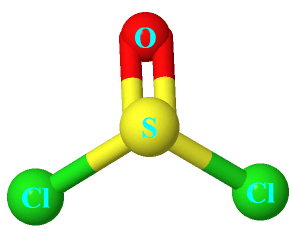Thionyl chloride appears as a colourless liquid is an inorganic compound with the chemical formula SOCl2. It has a suffocating pungent odour and is primarily used as a chlorinating agent. It is highly corrosive and toxic in nature.

In this article, we will discuss SOCl2 lewis structure, molecular geometry, bond angle, hybridization, polar or nonpolar, etc.

Thionyl chloride is used in the production of chemical weapons due to its high toxicity nature. Long term inhalation of this compound can cause many adverse health effects.

Properties of Thionyl chloride

• It appears as colourless to yellow fuming liquid
• It is soluble in water.
• It has a boiling point of 74.6 °C and a melting point of −104.5 °C.
• It has a dipole moment of 1.44D.
• It is non-flammable in nature.
 Name of Molecule Thionyl chloride Chemical formula SOCl2 Molecular geometry of SOCl2 Trigonal pyramid Electron geometry of SOCl2 Tetrahedral Hybridization Sp³ Total Valence electron for SOCl2 26 The formal charge of SOCl2 0
Page Contents

## How to draw lewis structure of SOCl2?

SOCl2 lewis structure contains a sulfur atom in central position whereas the two chlorine and one oxygen atom spread evenly around it. There is one double bond and two single bonds present in the lewis structure of SOCl2.

The lewis dot structure of SOCl2 disobeys the octet rule for attaining stability, let’s see how to draw this in a simple way.

## Steps to draw lewis structure of SOCl2

1. Count total valence electron in SOCl2

In the very first step, we will count the total valence electron in the SOCl2 molecule. To calculate the valence electron, look at the periodic group of the individual atoms – sulfur, oxygen, and chlorine.

The chlorine atom belongs to the periodic group 7A or 17th in the periodic table, hence, the valence electron in the chlorine atom is 7. The oxygen and sulfur atom are situated in the same periodic group which is 6A or 16th in the periodic table.

Hence, the valence electron in both sulfur and oxygen is 6.

⇒ Valence electron in chlorine atom = 7

⇒ Valence electron in oxygen atom = 6

⇒ Valence electron in sulfur atom = 6

∴ Total valence electron available for drawing the Lewis structure of SOCl2 = 6 + 6 + 7(2) = 26 valence electrons                [∴SOCl2 has one oxygen, one sulfur and two chlorine atoms]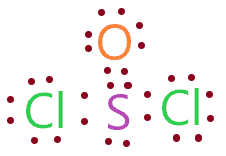2. Find the least electronegative atom and placed it at center

Now we will find the least electronegative atom in the SOCl2 compound, after that, we will place it at the center in the lewis diagram and the rest atoms will be spread around it.

The electronegativity value of the sulfur atom is 2.58, for a chlorine atom, it is 3.16. And for oxygen atoms, the electronegativity is 3.44.

Hence, the sulfur atom is the least electronegative atom in the SOCl2 compound, therefore, we will put the sulfur atom at a central position in the lewis diagram.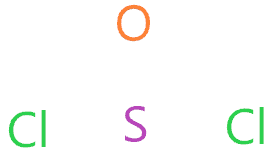3. Connect outer atoms to central atom with a single bond

In this step, we simply connect each outer atom(chlorine and oxygen) to the central atom(sulfur) with the help of a single bond.Now count the valence electron used in the above structure. A single bond means two electrons, in the above structure, three single bonds are used for connecting the two chlorine and one oxygen atom to the sulfur central atom.

Therefore, (3 single bonds × 2) = 6 valence electrons are used in the above structure from the total of 26 valence electrons available for drawing the lewis structure of SOCl2.

∴ (26 – 6) = 20 valence electrons

So, we are left with 20 valence electrons more.

4. Place remaining valence electrons starting from outer atom first

In this step, we will put the remaining valence electron on the outer atom first for completing their octet. In a SOCl2 molecule, the outer atom is oxygen and chlorine. And both these require 8 electrons in their outer shell to complete the octet.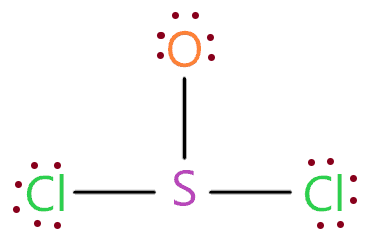As you see in the above figure, we have placed the 6 electrons represented as dots around each outer atom(chlorine and oxygen). This is because chlorine and oxygen atoms already have 2 electrons(one single bond) in their outer shell.

Hence, both these only require 6 more electrons for completing the octet.

Now once again count the total valence electron in the above structure.

∴ (3 single bond × 2 electrons + 18 electrons represented as dots) = 24 valence electrons are used in the above structure.

5. Complete central atom octet and make covalent bond if necessary

As we already completed the octet of outer atoms, now, we have to complete the octet of the central atom also which is sulfur in the SOCl2 compound.

In the 4th step structure, we used the 24 valence electrons from the total of 26 available valence electrons. Hence, we are left with only two valence electrons. Just put these electrons over the sulfur atom.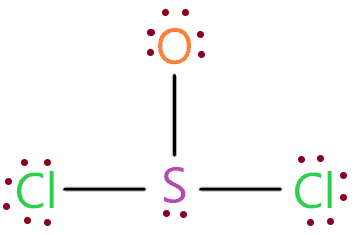So, we have used all the valence electrons that are available for drawing the lewis structure of SOCl2. But we don’t know yet if the above structure is stable or not.

For checking this we will move forward to the formal charge concept.

6. Check the stability with the help of a formal charge concept

The structure with the formal charge close to zero or zero is the best and stable lewis structure.

To calculate the formal charge on an atom. Use the formula given below-

⇒ Formal charge = (valence electrons – lone pair electrons –  1/2shared pair electrons)

We will calculate the formal charge on the 5th step structure to verify its stability.

For sulfur atom-

⇒ Valence electron of sulfur = 6

⇒ Lone pair electrons on sulfur = 2

⇒ Shared pair electrons around sulfur(three single bonds) = 6

∴ F.C. on sulfur atom = (6 – 2 – 6/2) = +1.

For oxygen atom –

⇒ Valence electron of oxygen = 6

⇒ Lone pair electrons on oxygen = 6

⇒ Shared pair electrons around oxygen(one single bond) = 2

∴ F.C. on oxygen atom = (6 – 6 – 2/2) = -1.

For chlorine atom –

⇒ Valence electron of chlorine = 7

⇒ Lone pair electrons on chlorine = 6

⇒ Shared pair electrons around chlorine(one single bond) = 2

∴ F.C. on chlorine atom = (7 – 6 – 2/2) = 0Obviously, the above structure is not stable as it attains a very high formal charge. We have to lower the formal charge of the above structure by converting lone pairs to bond pairs (covalent bonds).

Note: The sulfur atom is exceptional to the octet rule as it can hold more than 8 electrons in its outermost shell because it is the element of the period (row) 3 in the periodic table that can have expanded octet.

Expanded octet: A case where an atom shares more than eight electrons with its bonding partners.

The chlorine atom already getting the formal charge zero, hence, we must not try to touch it. Just convert the lone pair of oxygen atoms to the bonded pair(covalent bond).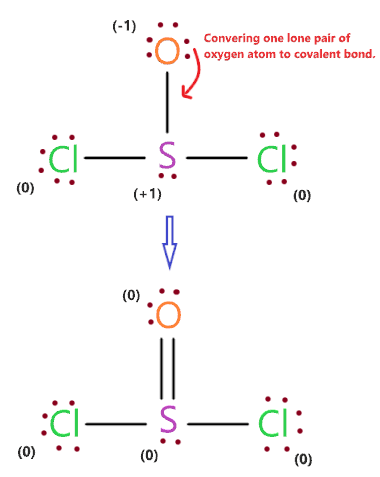As you see in the above figure, we convert the one lone pairs of oxygen atoms to a covalent bond and we successfully reduced the formal charge of sulfur and oxygen atom equal to zero

So, the final lewis structure of SOCl2 contains one double bond and two single bonds, the chlorine and oxygen atom have 8 electrons in their outer shell and the sulfur central atom has 10 electrons in its outer shell as it has the ability to expand the octet.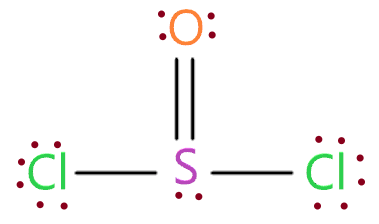### SOCl2 lewis dot structure

‍∴ The overall formal charge in SOCl2 is zero. Hence, the above lewis structure of thionyl chloride is most stable, appropriate, and reliable.

## What is the molecular geometry of SOCl2?

The molecular geometry of SOCl2 is a trigonal pyramid because the central atom of it has one lone pair and is attached to the three bonded pairs that will repel each other according to the VSEPR theory, hence, this electron cloud (lone pair and bond pair) tried to remain far as much as possible and in this process, they take up the position where the shape of SOCl2 appears as a trigonal pyramid.### SOCl2 molecular geometry

We can use another method for finding the electron and molecular geometry of SOCl2 which is the AXN method.

AXN notation for SOCl2 molecule:

• A represents the central atom, so, in the SOCl2 molecule, sulfur is the central atom. A = Sulfur
• X denotes the bonded atoms to the central atom, as we know, sulfur is bonded with two chlorine atoms and one oxygen atom. Therefore, X = 3
• N denotes the lone pair on the central atom, as per SOCl2 lewis structure, sulfur has only one lone pair. Hence, N = 1

So, the AXN notation for the SOCl2 molecule becomes AX3N1

As per the VSEPR chart, if a molecule central atom is attached with three bonded atoms and has contained only one lone pair on the central atom then the molecular geometry of that molecule is the trigonal pyramid in nature, and electron geometry is tetrahedral.

 Bonded atoms Lone pair Generic formula Hybridization Molecular geometry Electron geometry 1 0 AX S Linear Linear 2 0 AX2 Sp Linear Linear 1 1 AXN Sp Linear Linear 3 0 AX3 Sp² Trigonal planar Trigonal planar 2 1 AX2N Sp² Bent Trigonal planar 1 2 AXN2 Sp² Linear Trigonal planar 4 0 AX4 Sp³ Tetrahedral Tetrahedral 3 1 AX3N1 Sp³ Trigonal pyramid Tetrahedral 2 2 AX2N2 Sp³ Bent Tetrahedral 1 3 AXN3 Sp³ Linear Tetrahedral 3 2 AX3N2 Sp³d T-shaped Trigonal bipyramidal

Hence, the molecular shape of SOCl2 is a trigonal pyramid, and electron geometry is tetrahedral.

## What is the Hybridization of SOCl2?

We can calculate the hybridization of SOCl2 using the steric number formula given below:

∴ Steric number of SOCl2 = (Number of bonded atoms attached to sulfur + Lone pair on sulfur atom)

As per the SOCl2 lewis dot structure, the sulfur atom is bonded with two chlorine atoms and one oxygen atom and contains only one lone pair.

∴ Steric number of SOCl2 = (3 + 1) = 4

So, for the steric number of 4, we get the hybridization of SOCl2 is Sp3.

## What is the Bond angle of SOCl2?

The bond angle of ∠O = S – Cl is 107.4º and ∠Cl – S – Cl is = 96.5º.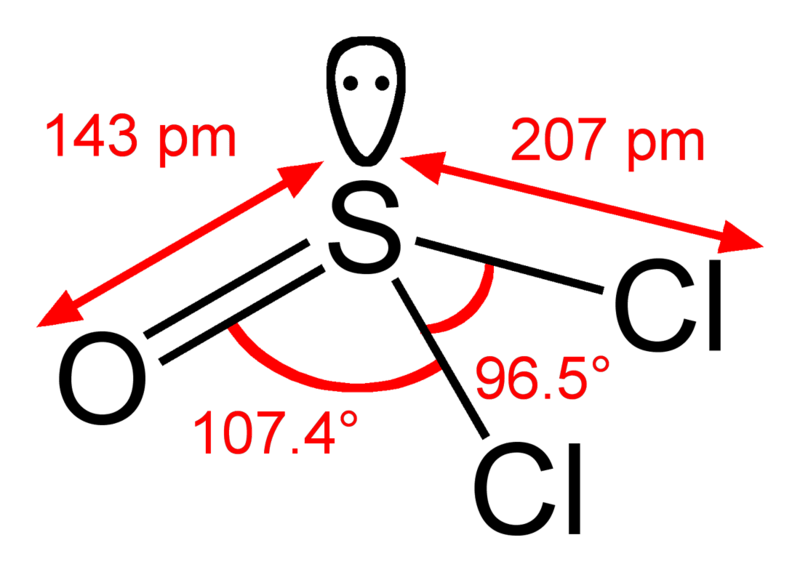## Thionyl chloride polarity: is SOCl2 polar or nonpolar

So, Is SOCl2 polar or nonpolar? SOCl2 is a polar molecule because of its asymmetrical structure that causes uneven charge distribution and that contributes to the nonzero dipole moment and making this molecule polar in nature.

Also, the sulfur atom gets the positive charge because of its less electronegativity and both chlorine and oxygen atom gets negative charge because of there high electronegativity.

The separation of positive and negative charges leads the dipole moment in SOCl2 directed from sulfur to chlorine or sulfur to oxygen.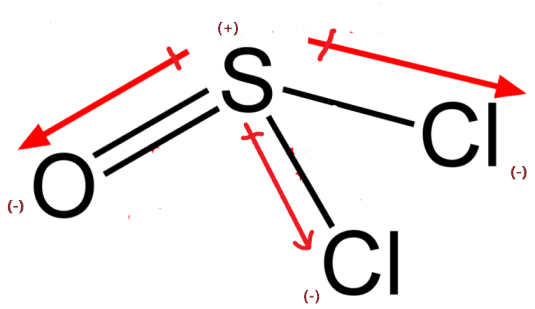The net dipole moment of SOCl2 is 1.44D.

## Summary

• The total valence electron available for drawing the SOCl2 lewis structure is 26.
• The hybridization of SOCl2 is Sp³.
• SOCl2 is a polar molecule and has a net dipole moment of 1.44D.
• The molecular geometry of SOCl2 is trigonal pyramidal and its electron geometry is tetrahedral.
• Lewis dot structure of SOCl2 contains two single bonds, one double bond, and one lone pair on the central atom.
##### Subscribe to Blog via Email

Join 2 other subscribers

Share it...